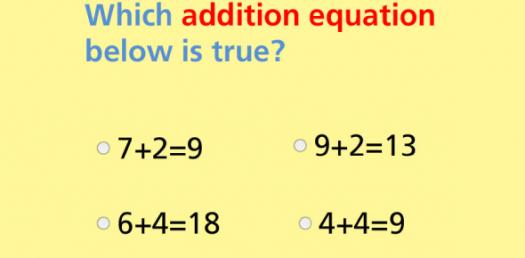48 Questions | Attempts: 2841SettingsIn this quiz, we look to give the person skills to solve mathematical problems most especially, addition easily. Addition is one of the most important concepts in mathematics and is probably the second thing after numbers that people learn. These questions will give you the skills necessary to improve anyone’s math skills. Try them out and come back to try others like it!

• 1.
3+0=
• 2.
2+2=
• 3.
1+1=
• 4.
1+2=
• 5.
3+1=
• 6.
2+2=
• 7.
3+0=
• 8.
3+1=
• 9.
3+0=
• 10.
2+0=
• 11.
1+1=
• 12.
2+1=
• 13.
1+3=
• 14.
1+2=
• 15.
3+1=
• 16.
2+1=
• 17.
4+0=
• 18.
2+2=
• 19.
1+1=
• 20.
3+1=
• 21.
2+0=
• 22.
3+0=
• 23.
1+2=
• 24.
3+0=
• 25.
1+3=

## Related TopicsBack to top
×

Wait!
Here's an interesting quiz for you.